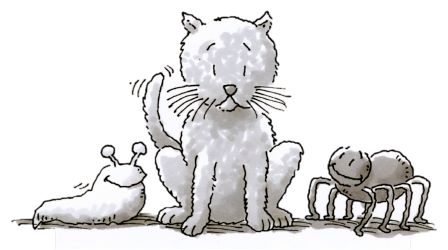### Home > MC1 > Chapter 4 > Lesson 4.1.1 > Problem4-7

4-7.

Complete each of following statements.

1. If one cat has $16$ whiskers, then seven cats will have ______ whiskers.

To solve this problem, your multiplication skills might come in handy.

2. If three slugs have six eye-stalks, then two slugs will have ______ eye-stalks.

Can you find how many eye-stalks one slug has? Maybe drawing a diagram can help!

Don't forget, reading the question multiple times can help make sure you understand what you are being asked. Here, you have to find how many eye-stalks two slugs will have.

3. If eight spiders have $64$ legs, then $5$ spiders will have ______ legs.

First, you will need to find how many legs one spider has. Can you use division to help?

After completing the first step, use multiplication to find how many legs $5$ spiders will have.

$5$ spiders will have $40$ legs.